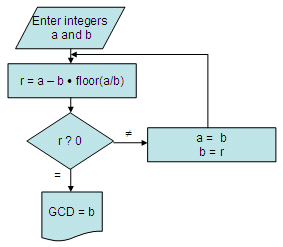# Euclidean Algorithm

This program calculates the Greatest Common Denominator (GCD) of two integers. It is based on the Euclidean algorithm for finding the GCD.Basic Algorithm - Flow chart

This is the full Matlab program that follows the flow-chart above, without using the built-in 'gcd' instruction.

% Clears screen and deletes all the variables in the workspace
clear; clc

% Asks the user for input and takes only positive numbers into account
a = input('First number: ');
b = input(
'Second number: ');
a = abs(a);
b = abs(b);

% This is the real trick, normally performed a number of times
r = a - b*floor(a/b);

% Repeats the operation until updates of a equal updates of b
while r ~= 0
a = b;
b = r;
r = a - b*floor(a/b);

end

% Displays the result
GCD = b

Example 1:

Find the greatest common denominator of 18 and 50.

Run the algorithm above and enter data:

First number: 18
Second number: 50

GCD =
2

The built-in Matlab command 'gcd' also works on vectors. For example:

a = [50 150 20]
b = [18 115 5]

>> gcd(a,b)

ans =
2     5     5

From 'Euclidean Algorithm' to home

From 'Euclidean Algorithm' to Matlab Cookbook